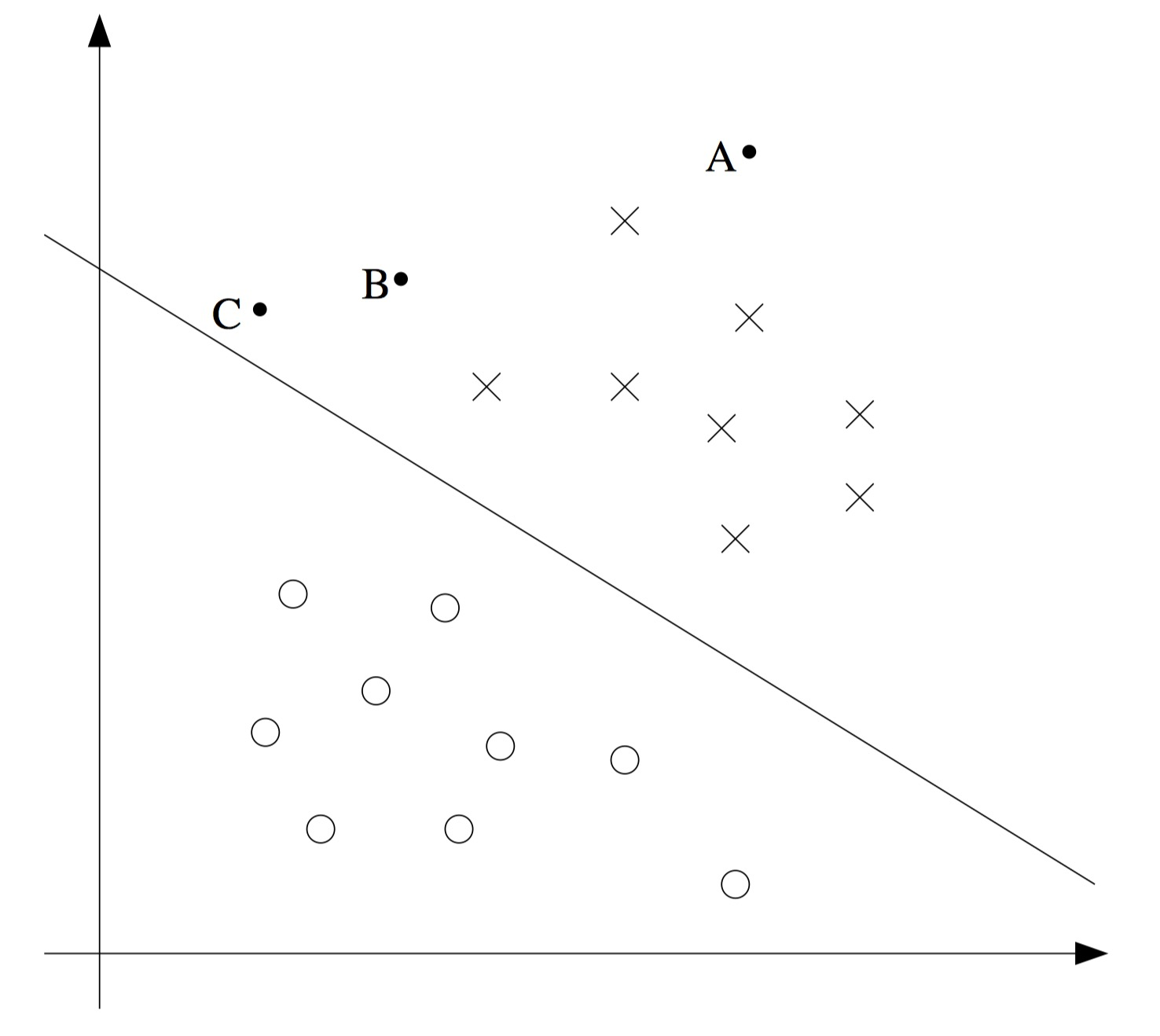## BGD 和 SGD 的区别？SGD 为什么会收敛？

BGD 是 batch gradient descent ，每次更新 θ 值都需要用到整个训练集，直到 θ 收敛到某一个数；SGD 是 stochastic gradient descent ，每次更新 θ 只需要用到训练集中的一组数据，所以其优势也直观地体现出来了：快。那么为什么在 SGD 的方法下，θ的值能够收敛，也就是 cost function 相对于 θ 的导数可以收敛呢？BGD 的情况比较容易理解，因为每次所选的数据都是一样的；但 SGD 每次都是随机选的一组数据…

## 正规方程（normal equations）是怎么来的？

$$J\left ( \theta \right ) = \frac{1}{2} \sum_{i=1}^{m}\left ( h_{\theta} \left ( x^{\left ( i \right )} \right ) - y^{\left ( i \right )}\right )^{2} = \frac{1}{2} \left ( X\theta - \vec{y} \right )^{T} \left ( X\theta - \vec{y} \right )$$

\begin{equation}\begin{split}
\bigtriangledown_{\theta}J\left ( \theta \right )
&= \bigtriangledown_{\theta} \frac{1}{2} \left ( X\theta - \vec{y} \right )^{T} \left ( X\theta - \vec{y} \right ) \\
&= \frac{1}{2} \bigtriangledown_{\theta} \left ( \theta^{T}X^{T}X\theta - \theta^{T}X^{T}\vec{y} - \vec{y}^{T}X\theta + \vec{y}^{T}\vec{y}\right ) \\
&= \frac{1}{2} \bigtriangledown_{\theta} tr\left ( \theta^{T}X^{T}X\theta - \theta^{T}X^{T}\vec{y} - \vec{y}^{T}X\theta + \vec{y}^{T}\vec{y}\right ) \\
&= \frac{1}{2} \bigtriangledown_{\theta} \left ( tr\theta^{T}X^{T}X\theta - 2 tr \vec{y}^{T}X\theta \right ) \\
&= \frac{1}{2} \left ( X^{T}X\theta + X^{T}X\theta - 2X^{T}\vec{y} \right ) \\
&= X^{T}X\theta - X^{T}\vec{y}
\end{split}\end{equation}

## 逻辑回归损失函数的说明推导

\begin{equation}\begin{split}
L(\theta)
&= p(\overrightarrow{y}|X;\theta) \\
&= \prod_{i=1}^{m} p(y^{(i)}|x^{(i)};\theta) \\
&= \prod_{i=1}^{m} (h_\theta (x^{(i)}))^{y^{(i)}} (1-h_\theta (x^{(i)}))^{1-y^{(i)}} \end{split}\end{equation}

\begin{equation}\begin{split}
\frac{\partial}{\partial \theta_j} l(\theta)
&= (y\frac{1}{g(\theta^T x)} - (1-y)\frac{1}{1-g(\theta^T x)})\frac{\partial}{\partial \theta_j}g(\theta^T x) \\
&= (y\frac{1}{g(\theta^T x)} - (1-y)\frac{1}{1-g(\theta^T x)})g(\theta^T x)(1-g(\theta^T x))\frac{\partial}{\partial \theta_j}\theta^T x \\
&= (y(1-g(\theta^T x)) - (1-y)g(\theta^T x)) x_j \\
&= (y - h_\theta(x)) x_j
\end{split}\end{equation}

$$\theta_j := \theta_j + \alpha (y^{(i)} - h_\theta(x^{(i)}))x_j^{(i)}$$

## SVM 说明与核心推导

SVM (Support Vector Machine) 即 支持向量机 ，其学习目标是使得分离超平面与支持向量（离超平面最近的向量）的距离最大化，也即求出分离超平面 （表示为 wx + b = 0 ）对应的 w 和 b ，这可以形式化为一个凸二次规划的求解问题。因此，SVM 学习算法的核心/本质是求解凸二次规划的最优化算法。$$\gamma_{i} = y_{i} \left ( \frac{w}{||w||}\cdot x_{i} + \frac{b}{||w||}\right )$$

\begin{equation}\begin{split}
& \underset{w,b}{max} \quad \gamma \\
& s.t. \quad y_{i} \left ( \frac{w}{||w||}\cdot x_{i} + \frac{b}{||w||}\right ) \geqslant \gamma, \quad i = 1,2,…,N
\end{split}\end{equation}

\begin{equation}\begin{split}
& \underset{w,b}{max} \quad \frac{\widehat{\gamma}}{||w||} \\
& s.t. \quad y_{i} \left ( w \cdot x_{i} + b \right ) \geqslant \widehat{ \gamma }, \quad i = 1,2,…,N
\end{split}\end{equation}

\begin{equation}\begin{split}
& \underset{w,b}{min} \quad \frac{1}{2} ||w||^{2} \\
& s.t. \quad y_{i} \left ( w \cdot x_{i} + b \right ) - 1 \geqslant 0, \quad i = 1,2,…,N
\end{split}\end{equation}